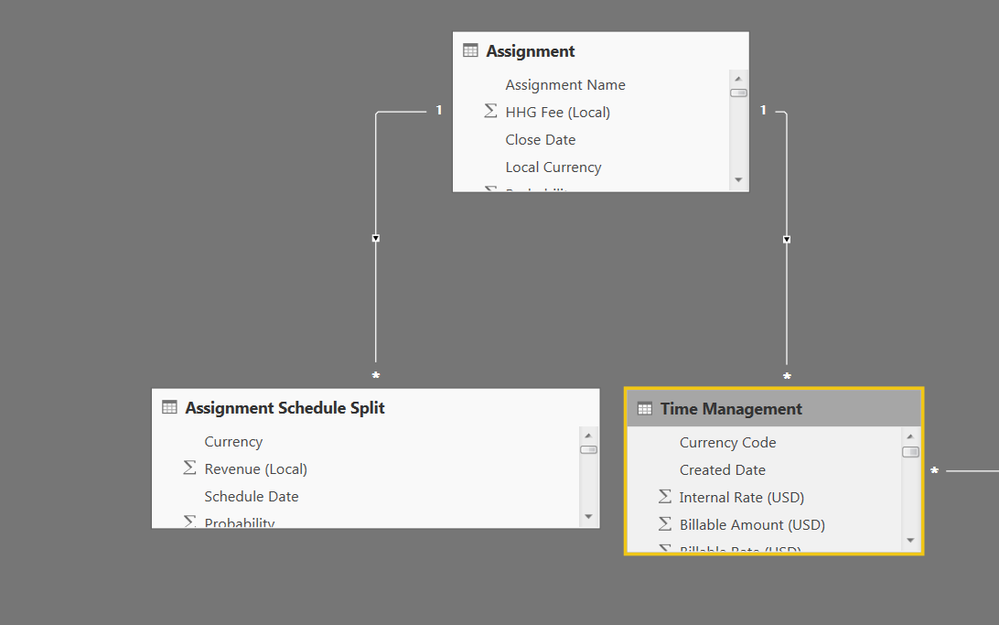cancel
Showing results for
Did you mean:Helper I

## Sum from related table

I am trying to sum values that are on other tables onto a master table, but I can't seem to get it to work.  It is essentially a Sumif function, but I can't seem to get Calculate and or SUMX to work.  It always wants to return the fee for the entire related table (Revenue or Time).

Please see the below image.  I am trying to sum Fees and Hours onto the Assignments table for the individual assignments.  The Revenue and Time tables are linked to Assignments.1 ACCEPTED SOLUTIONAnonymous
Not applicable

Try:

AssignmentTable[Total Fee] = CALCULATE(SUM(Revenue[Fee]))

The calculate is weird and required.

11 REPLIES 11Helper II

Best to use power query/query editor then bring the data through in one table.

Start with 'group by' function which is basically a sumif and do this for both the revenue table and the time table. This will produce one summed up value for each assignment. Then merge the time table into the revenue table, linking them by the assignment, select the hours colum to bring through to the revenue table. This will produce what you need 3 rows and 2 columns of data. Boom!Anonymous
Not applicable

Your "What is Happening" is confusing on the Assignment table -- I assume there are actually 3 rows there?Helper I

Sorry. It isn't summing up at the relevant rows.  It is just giving me a total for all assignments.  I would like the total fees and hours for each assignment based on the other tables.Helper I

You can disregard the bottom half. I am just trying to create the Fee and Hours columns in the Assignment TableAnonymous
Not applicable

Try:

AssignmentTable[Total Fee] = CALCULATE(SUM(Revenue[Fee]))

The calculate is weird and required.New Member

What about a SUMIFS formula? if you want to also exclude a category from the sumif formula?Helper I

Didn't work.  It seems to just calculate the total rather than for each assignment.  I tried both as a measure and as a column.Anonymous
Not applicable

Can you show a picture of your model relationships -- this should have worked fine...Helper I

Structure:Relationships: The tables are connected via an Assignment Key field.Formula: HHG  = CALCULATE(SUM('Assignment Schedule Split'[Revenue (USD)])).

- The fields on the left of HHG are from the Assignment table.

- The formula is on the assignments tableHelper I

I fixed it.  You were right on the formula.  The "key" field was slightly different, which caused all the issues.

Thanks!Helper I

I feel that this one is asking the same question (I have a one to many relationship), but i havent been able to get it to work http://community.powerbi.com/t5/Desktop/Create-a-Calculated-Column-from-Different-Tables/m-p/60773#M...